# Create Dynamic Hyperlinks in Excel

This tutorial will demonstrate how to create dynamic hyperlinks in Excel Sheet.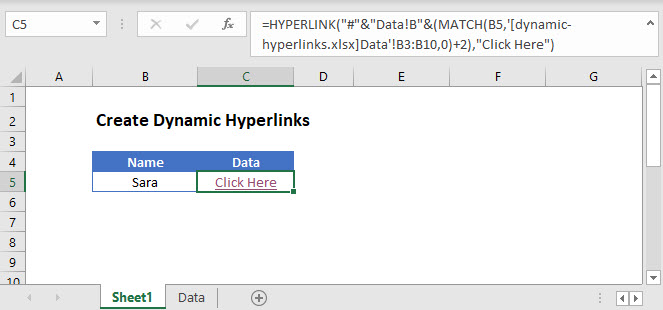In the example below, we will create a dynamic hyperlink to a cell based on the result of a MATCH Function.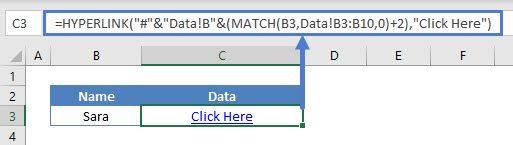Here we lookup “James” in sheet ‘Data’ and link to corresponding row. Let’s break the function up into two parts to make it easier to follow:

The HYPERLINK Function allows you to create a link to a location point in the same workbook, different workbook, or an external URL. Its syntax is: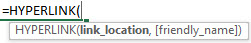The following example will dynamically link to cell B8 in the worksheet ‘Data‘.

``=HYPERLINK("#"&"Data!B"&C3,"Click Here")``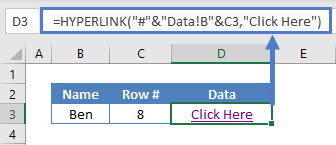In the above example, the ‘Row #‘ column provides the row number for the ‘link_location‘ argument. It has been calculated using the MATCH Function like this:

``=MATCH(B3,Data!B3:B10,0)+2``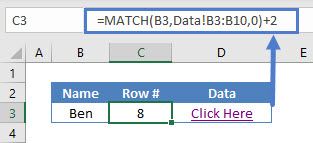Note: To get the required row number, the number of rows above the selected range (2) has been added.

### Excel Practice Worksheet

Practice Excel functions and formulas with our 100% free practice worksheets!